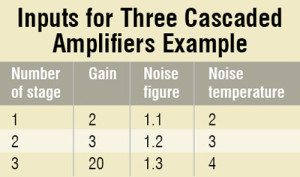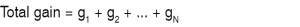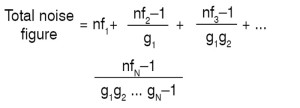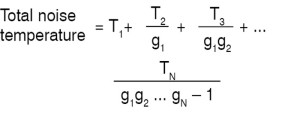Sunday, December 10, 2023

# Gain and Noise Calculations of Cascaded Systems Using MATLAB

Parameters like noise, signal-to-noise ratio and gain play an important role in the performance of communication systems. Here we explain these terms and their calculation using MATLAB (matrix laboratory) software. -- Anupriya AsthanaParameters like noise, signal-to-noise ratio and gain play an important role in the performance of communication systems. Here we explain these terms and their calculation using MATLAB (matrix laboratory) software.

## Gain and noise basics

Gain is a measure of the ability of a circuit (often an amplifier) to increase the power or amplitude of a signal from the input to the output. Noise deteriorates the quality of the received signal (in case of analogue systems) and degrades the throughput (in case of digital systems). Therefore it is important to maximise signal-to-noise ratio (SNR) in a communication system.

The noise figure (NF) is a measure of the degradation of the SNR. In cascaded systems, total noise factor depends upon the gain and NF of each block (or stage). For example, in the case of amplifiers connected in cascade, each amplifier will add its own noise. Hence the overall noise factor will be due to the contribution by each amplifier.

Fig. 1 shows the cascade connection of two amplifiers with noise figures f1 and f2 and gains g1 and g2, respectively.

The noise contribution of each successive stage in a cascaded system is smaller and smaller. The noise of the first stage is the most important. Thus every communication system employs a low-noise amplifier (LNA) at the front to relax the noise requirements. A typically good LNA may have gain of 20 dB and NF less than 1.5 dB.

Noise figure provides a simple method to determine the impact of system noise on sensitivity. Noise temperature is used to express the level of available noise power introduced by a component or source.

## Software programIn this project, MATLAB software is used to design a calculator for the calculation of noise factors. Such type of calculators can be easily created by playing with the array and matrix properties of MATLAB, and can be used for any cascade system with any number of stages. Coding is developed by using simple commands (without using any typical command from MATLAB library).

For a better understanding of this project, it is suggested that you first go through its flow-chart (refer Fig. 2) and then try to understand its programming. In programming, all factors are calculated one by one. First of all, the total gain of the cascade system is calculated. Then by using Friis’s formula for noise, the total noise figure and noise temperature are calculated.

Amplifiers are selected as components of a cascade system. The block diagram of a cascade system is shown in Fig. 3.

Total gain of the cascade system is given by:where g1, g2, … , gN are gains of the cascaded system.

Cascaded noise figure in expanded form is given by:where nf1, nf2, … , nfN are noise figures of the cascaded system.

Cascaded noise temperature in expanded form is given by:where T1, T2, … , TN are noise temperatures of the cascaded system.

### MATLAB source code

clc

x=input(‘No of stages,x:’);

%enter gain of all amplifiers in
array format
g=input(‘enter gain of all amplifiers
in array format:’);

%enter noise figure of 1st amplifier
nf1=input (‘enter noise figure of 1st
amplifier:’);
noisefactor=0;
gn=1;
TOTAL_GAIN=0;

for i=1:x
TOTAL_GAIN=TOTAL_GAIN+g(i);
end
for i=2:x
a=g(i-1);
b(i-1)=[a];
end
for i=1:(x-1)
b(i);
end
for i=1:(x-1)
noisefigure=input(‘enter noise figure
of next amplifier:’);
N=noisefigure-1;
gn=gn*b(i);
NF=N/gn;
noisefactor=noisefactor+NF;
end

%total noise factor of the cascaded
system is
TOTAL_NOISEFACTOR=nf1+noisefactor;

T1=input (‘enter noise temp of 1st
amplifier:’);
noisetemp=0;
gn=1;
for i=2:x
a=g(i-1);
b(i-1)=[a];
end
for i=1:(x-1)
b(i);
end
for i=1:(x-1)
T=input(‘enter noise temp of next
amplifier:’);
gn=gn*b(i);
NT=T/gn;
noisetemp=noisetemp+NT;
end
%total noise temp of the cascaded
system is
TOTAL_TEMPERATURE=T1+noisetemp;

%results
TOTAL_GAIN
TOTAL_NOISEFACTOR
TOTAL_TEMPERATURE

## Example

Let us calculate gain, noise figure and noise temperature of three cascaded amplifiers (Fig. 4) by using the above-mentioned MATLAB program. The inputs to be entered by the user are shown in the table for easy understanding.

The output of the program is:

No of stages,x:3
enter gain of all amplifiers in array
format:[2 3 20]
enter noise fig of 1st amplifier:1.1
enter noise figure of next
amplifier:1.2
enter noise figure of next
amplifier:1.3
enter noise temp of 1st amplifier:2
enter noise temp of next amplifier:3
enter noise temp of next amplifier:4

TOTAL_GAIN= 25
TOTAL_NOISEFACTOR= 1.2500
TOTAL_TEMPERATURE= 4.1667

The author is an M.Tech student at Galgotias University in Greater Noida, UP

#### This project was first published on 23 December 2015 and was updated on 7 July 2020.

1.David

Help us where to write this program ?

•EFY Team

2.suma

•EFY Team

Hi, we were facing some issues earlier with the Source page. Kindly refresh the page and retry.